Question

# 1. Given a binary code, determine the number of errors that it can detect and the...

1. Given a binary code, determine the number of errors that it can detect and the number of errors that it can correct. WRITE A PROGRAM PYTHON or JAVA. both would be nice

package Networking;

import java.util.*;

public class ErrorDectCorrect

{

static int setParityBit(int data[])

{

// Initializing count to zero which will count the number of 1.

int counter = 0;

// Loops till data length

for(int c = 0; c < data.length; ++c)

// Increase count if value in array "data" is 1.

if(data[c] == 1)

++counter;

// Returns 0 if counter value is even number (even number of 1s)

if((counter % 2) == 0)

return 0;

// Otherwise returns 1 for odd number of 1

else

return 1;

}// End of method

// main method definition

public static void main(String args[])

{

// Scanner class object created

Scanner keyboard = new Scanner(System.in);

System.out.print("\n Error detection and correction using EVEN parity");

// Sets number of data bits

int numberOfDataBits = 4;

// Accepts 4 bits of data

System.out.print("\n\n Enter 4 data bits: ");

// Declares an array to accept data bits

int dataBits[] = new int;

for(int pos = numberOfDataBits - 1; pos >= 0; --pos)

{

System.out.print("Enter the value of D" + (pos + 1) + " -> ");

dataBits[pos]=keyboard.nextInt();

}// End of for loop

// Formula (2 ^ numberOfParityBits >= numberOfDataBits + numberOfParityBits + 1)

// Initializing it to zero.

int numberOfParityBits = 0;

// Calculating the value of k(number of parity bits).

while(Math.pow(2, numberOfParityBits) < (numberOfDataBits + numberOfParityBits + 1))

++numberOfParityBits;

System.out.println("\n" + numberOfParityBits +

" parity bits are required for the " +

"transmission of data bits.");

// Declares an array to store parity bits

int parityBits[] = new int[numberOfParityBits];

// Array to store the hamming code.

// (n + numberOfParityBits + 1) as we start from pos 1.

int hammingCode[] = new int[numberOfDataBits + numberOfParityBits + 1];

// Initialize each hammingCode array element to -1

for(int pos = 0; pos < 7; ++pos)

hammingCode[pos] = -1;

int count = 0;

int c = 2;

while(count < 4)

{

++c;

if(c == 4)

continue;

hammingCode[c] = dataBits[count];

++count;

}// End of while loop

int p1[] = {hammingCode, hammingCode, hammingCode, hammingCode};

int p2[] = {hammingCode, hammingCode, hammingCode, hammingCode};

int p3[] = {hammingCode, hammingCode, hammingCode, hammingCode};

int parity[] = new int;

// Calls the method to set the value of parity bit

parity= setParityBit(p1);

parity= setParityBit(p2);

parity= setParityBit(p3);

// Inserting the parity bits in the hamming code

hammingCode = parity;

hammingCode = parity;

hammingCode = parity;

System.out.println("\n\n SENDER SIDE:");

System.out.print("\n The data bits entered are: ");

for(int pos = 3; pos >= 0; --pos)

System.out.print(dataBits[pos] +" ");

System.out.println("\n\n The Parity bits are: ");

for(int pos = 2; pos >= 0; --pos)

System.out.println("Value of P " + (pos + 1) + " is " + parity[pos] + " ");

System.out.print("\n\n The Hamming code is as follows :-" +

"\nD4 D3 D2 P3 D1 P2 P1 \n");

for(int pos = (numberOfDataBits + numberOfParityBits); pos > 0; --pos)

System.out.print(hammingCode[pos] + " ");

System.out.println();

System.out.println("\n Enter the hamming code with error at " +

"any position of your choice." +

"\nNOTE: ENTER A SPACE AFTER EVERY BIT POSITION." +

"\nError should be present only at one bit position");

for(int pos = 7; pos > 0; --pos)

hammingCode[pos] = keyboard.nextInt();

int p4[]={hammingCode, hammingCode, hammingCode, hammingCode};

int p5[]={hammingCode, hammingCode, hammingCode, hammingCode};

int p6[]={hammingCode, hammingCode, hammingCode, hammingCode};

parity= setParityBit(p4);

parity= setParityBit(p5);

parity= setParityBit(p6);

int position = (int)(parity * Math.pow(2,2) +

parity * Math.pow(2,1)+

parity * Math.pow(2,0));

System.out.print("\n Error is detected at position " + position +

" at the receiving end.");

System.out.print("\n Correcting the error.... ");

if(hammingCode[position] == 1)

hammingCode[position]=0;

else

hammingCode[position] = 1;

System.out.print("\n The correct code is ");

for(int pos = 7; pos > 0; --pos)

System.out.print(hammingCode[pos] + " ");

}// End of main method

}// End of class

Sample Output:

Error detection and correction using EVEN parity

Enter 4 data bits: Enter the value of D4 -> 1
Enter the value of D3 -> 0
Enter the value of D2 -> 1
Enter the value of D1 -> 0

3 parity bits are required for the transmission of data bits.

SENDER SIDE:

The data bits entered are: 1 0 1 0

The Parity bits are:
Value of P 3 is 0
Value of P 2 is 1
Value of P 1 is 0

The Hamming code is as follows :-
D4 D3 D2 P3 D1 P2 P1
1 0 1 0 0 1 0

Enter the hamming code with error at any position of your choice.
NOTE: ENTER A SPACE AFTER EVERY BIT POSITION.
Error should be present only at one bit position
1 0 1 1 0 0 1

Error is detected at position 7 at the receiving end.
Correcting the error....
The correct code is 0 0 1 1 0 0 1

#### Earn Coins

Coins can be redeemed for fabulous gifts.

Similar Homework Help Questions
• ### Given two binary tree, can you determine if they are identical or not? Write a program...

Given two binary tree, can you determine if they are identical or not? Write a program for that. Algorithm: a) If both trees are NULL then return true b) If both trees are not NULL then, return TRUE if they are identical. In java.

• ### 1. (30 points) Consider the systematic binary linear (6,3) code with generator matrix 1 0 01...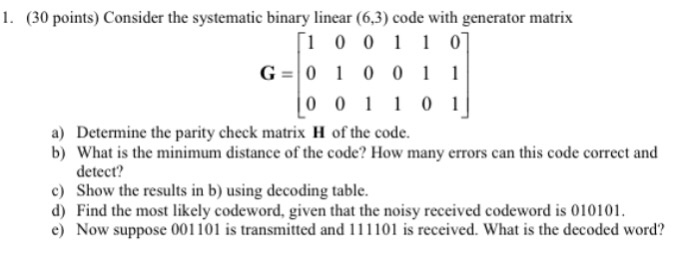1. (30 points) Consider the systematic binary linear (6,3) code with generator matrix 1 0 01 1 0 G- 0 1 0 0 1 1 a) Determine the parity check matrix H of the code. b) What is the minimum distance of the code? How many errors can this code correct and detect? c) Show the results in b) using decoding table d) Find the most likely codeword, given that the noisy received codeword is 010101. e) Now suppose 001101...

• ### In C:Write a program that can determine whether a user input is a binary number or...

In C:Write a program that can determine whether a user input is a binary number or not. (1, 2, 3, 8, 13) Write a program that accomplishes all of the following: Greets the user and informs them that the program will determine whether an input from the user is a valid binary number or not (e.g., consisting of only 0’s and/or 1’s). Accept an integer input from the user. Assume that the user provides a valid numeric input that will...

• ### Assignment Modify the code Binary File IO Example Code. The code reads and prints information abo...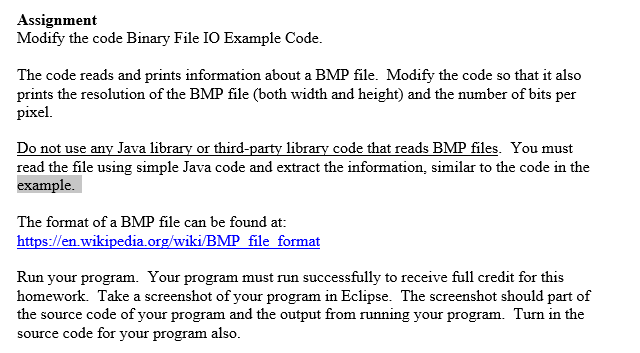In java Assignment Modify the code Binary File IO Example Code. The code reads and prints information about a BMP file. Modify the code so that it also prints the resolution of the BMP file (both width and height) and the number of bits per pixel. Do not use any Java library or third-party library code that reads BMP files. You must read the file using simple Java code and extract the information, similar to the code in the example....

• ### JAVA: Write a program that inputs a string that represents a binary number. The string can...

JAVA: Write a program that inputs a string that represents a binary number. The string can contain only 0s and 1s and no other characters, not even spaces. Validate that the entered number meets these requirements. If it does not, display an error message. If it is a valid binary number, determine the number of 1s that it contains. If it has exactly two 1s, display "Accepted". Otherwise, display "Rejected". All input and output should be from the console.

• ### CODE IN JAVA** V. Given a pointer to the root of a binary tree and a...

CODE IN JAVA** V. Given a pointer to the root of a binary tree and a pointer ‘p’ to a given node in the tree and a second pointer ‘q’ to another node in the tree write a routine which will return the total number of nodes in the tree that are on level ‘p’ and ‘q’. If they are on the same level you should multiply the answer by 3.

• ### Write an assembly program to translate an input number to its binary representation

Please help me out, i appreciate any help given. Oh and please if you have an answer write it out in assembly/sparc and not java or c++Your program should repeatedly prompts the user for an input and displaythe input in binary form, until the user chooses to quit.Note: For positive number, do not show leading zeros..Here is what the user will see when the program is executed:This Program prints the binary equivalent of a decimal numberPlease enter a number:78Binary number...

• ### A magnetic tape storing information in binary form has been corrupted, so it can only be...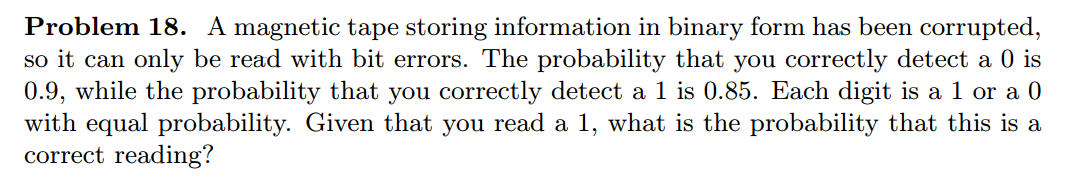A magnetic tape storing information in binary form has been corrupted, so it can only be read with bit errors. The probability that you correctly detect a 0 is 0.9, while the probability that you correctly detect a 1 is 0.85. Each digit is a 1 or a 0 with equal probability. Given that you read a 1, what is the probability that this is a correct reading?

• ### Can you send the code and the screenshot of it running? 1) Create a PYHW2 document...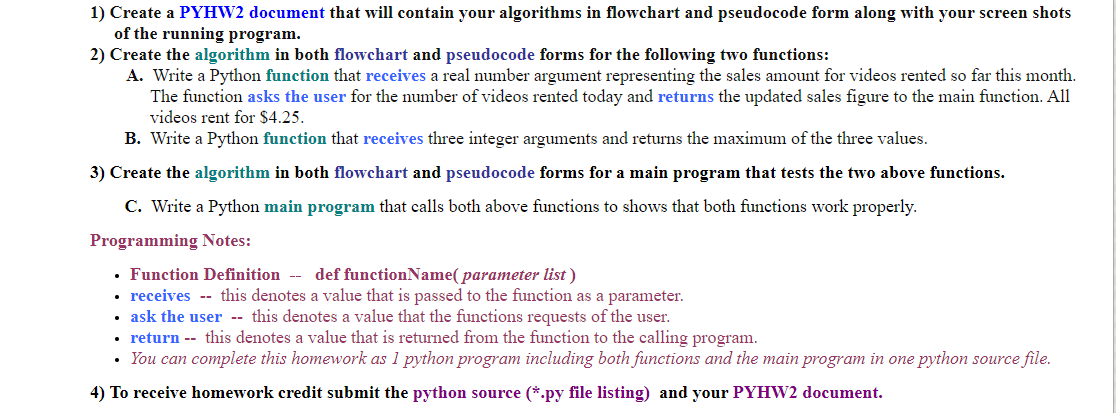Can you send the code and the screenshot of it running? 1) Create a PYHW2 document that will contain your algorithms in flowchart and pseudocode form along with your screen shots of the running program. 2) Create the algorithm in both flowchart and pseudocode forms for the following two functions: A. Write a Python function that receives a real number argument representing the sales amount for videos rented so far this month The function asks the user for the number...

• ### (9) Define a generating matrix G for a group code to be equal to the fol- lowing 3 x 13 binary matrix: 1001010101010 0100101010101 0011100110011 (a) List all the code words (b) What is the maximum nu...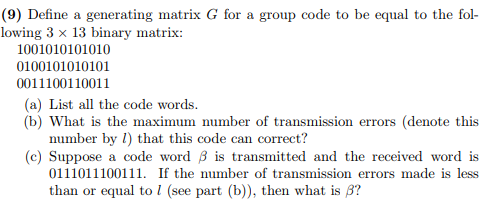(9) Define a generating matrix G for a group code to be equal to the fol- lowing 3 x 13 binary matrix: 1001010101010 0100101010101 0011100110011 (a) List all the code words (b) What is the maximum number of transmission errors (denote this number by 1) that this code can correct? (c) Suppose a code word β is transmitted and the received word s 0111011100111. If than or equal to (see part (b)), then what is B? the number of transmission...